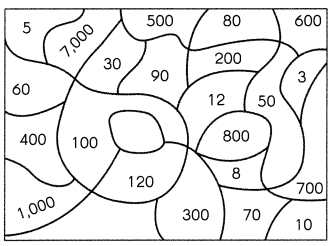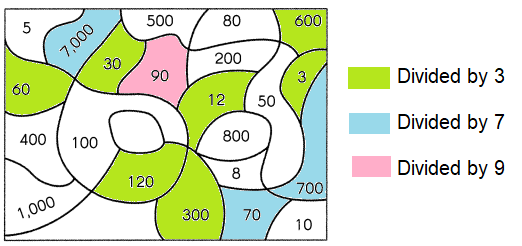# Math in Focus Grade 5 Chapter 2 Practice 5 Answer Key Dividing by Tens, Hundreds, or Thousands

Go through the Math in Focus Grade 5 Workbook Answer Key Chapter 2 Practice 5 Dividing by Tens, Hundreds, or Thousands to finish your assignments.

## Math in Focus Grade 5 Chapter 2 Practice 5 Answer Key Dividing by Tens, Hundreds, or Thousands

Complete.

Question 1.
100 ÷ 10 = _10__
10,

Explanation:
100 ÷ 10 = 10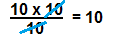100 is divided by 10 and the result is 10.

Question 2.
670 ÷ 10 = _67__
67,

Explanation:
670 ÷ 10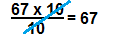670 is 10 times 67,
670 is 67 x 10.

Question 3.
1,050 ÷ __ = 105
105,

Explanation: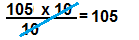1,050 is 105 x 10,
1,050 is divided by 10 and the result is 105.

Question 4.
_1,974__ ÷ 10 = 1,974
19,740,

Explanation: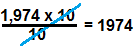19,740 can be written as 1974 x 10
it can be divided by 10 and the result is 1,974.

Question 5.
52,260 ÷ 10 = _5,260__
5,260,

Explanation: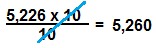5,2260 can be written as 5,226 x 10 it can be divided by 10 and
the result is 5,2260.

Question 6.
30,500 ÷ _10_ = 3,050
3,050,

Explanation: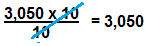3,050 can be written as 305 x 10 it can be divided by 10 and
the result is 3,050.

Complete.

5,610 ÷ 30
= (5,610 ÷ 10) ÷ 3
= 561 ÷ 3
= 187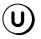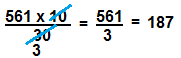Question 7.
3,000 ÷ 60
= (3,000 ÷ 10) ÷ _6__
= _300__ ÷ 6
= __50__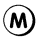50,

Explanation: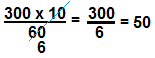= 3,000 ÷ 10÷ 6
= 300÷ 6
= 50.

Question 8.
1,040 ÷ 40
= (1,040 ÷ __10_) ÷ _4__
= _104__ ÷ __4__
= __26__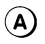26,

Explanation:
1,040 ÷ 40,
= 1,040 ÷ 10÷ 4
= 104 ÷ 4
= 26.

Complete.

Question 9.
8,700 ÷ 60
= (8,700 ÷ _10_) ÷ __6_
= _870__ ÷ _6__
= __145__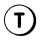145,

Explanation:
8,700 ÷ 60,
= 8,700 ÷ 10 ÷ 6,
= 870 ÷ 6,
= 145.

Question 10.
3,450 ÷ 50
= (3,450 ÷ _10_) ÷ _5__
= _345___ ÷ __5_
= __69__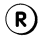69,

Explanation:
3,450 ÷  50,
= 3,450 ÷ 10 ÷ 5
= 345 ÷ 5
= 69.

Question 11.
34,230 ÷ 70
= (34,230 ÷ _10__) ÷ _7_
= _3,423__ ÷ _7__
= __489__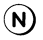489,

Explanation:
34,230 ÷ 70,
= 34,230 ÷ 10 ÷ 7,
= 3,423 ÷ 7,
= 489.

Which U.S. president had a sign on his desk that said ‘The buck stops here’?
Write the letters on pages 47 and 48 that match the answers below to find out.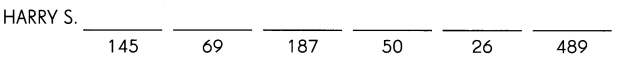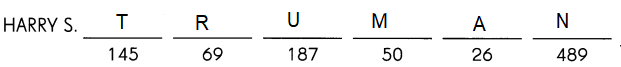Explanation:
The solutions from the above questions from 6 to 11,
according to the answers arrange the given alphabets to find out the word.

Divide.

Question 12.
3,400 ÷ 100 = _34__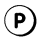34,

Explanation:
3,400 ÷ 10 ÷ 10,
= 340 ÷ 10,
= 34.

Question 13.
560,000 ÷ 1,000 = _560__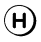560,

Explanation:
560,000 ÷ 1,000,
= 560,000 ÷ 10 ÷ 10 ÷ 10,
= 56,000 ÷ 10 ÷ 10,
= 5,600 ÷ 10,
= 560.

Question 14.
5,000 ÷ 100 = _50_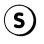50,

Explanation:
5,000 ÷ 100,
= 5,000 ÷ 10 ÷ 10,
= 500 ÷ 10,
= 50.

Question 15.
38,000 ÷ 1,000 = __38_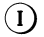38,

Explanation:
38,000 ÷ 1,000,
= 38,000 ÷ 10 ÷ 10 ÷ 10,
= 3800 ÷ 10 ÷ 10,
= 380 ÷ 10,
= 38.

Question 16.
7,700 ÷ 102 = _77__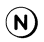77,

Explanation:
As 100 = 102,
= 7,700 ÷ 102,
= 7,700 ÷ 10 ÷ 10,
= 770 ÷ 10,
= 77.

Question 17.
360,000 ÷ 103 = _360__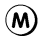360,

Explanation:
1000 = 103,
= 360,000 ÷ 10 ÷ 10 ÷ 10,
= 36,000 ÷ 10 ÷ 10,
= 3,600 ÷ 10,
= 360.

Question 18.
2,000 ÷ 102 = _20__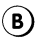20,
Explanation:
100 = 102,
2,000 ÷ 102,
= 2,000 ÷ 10 ÷ 10,
= 200 ÷ 10,
= 20.

Question 19.
415,000 ÷ 103 = _415__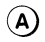415,

Explanation:
1000 = 103,
= 415,000 ÷ 10 ÷ 10 ÷ 10,
= 41,500 ÷ 10 ÷ 10,
= 4150 ÷ 10,
= 415.

To which class of animals does the salamander belong?
Write the letters that match the answers below to find out.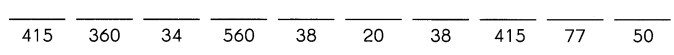AMPHIBIANS,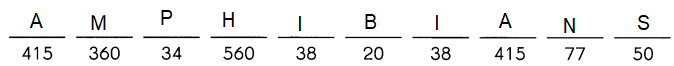Explanation:
The solutions from the above questions from 12 to 19,
according to the answers arrange the given alphabets to find out the word.

Question 21.
81,000 ÷ 900
= (81,000 ÷ _100_) ÷ __9_
= _810__ ÷ _9__
= _90___
90,

Explanation:
= 81,000 ÷ 900,
= 81,000 ÷ 100 ÷ 9,
= 81,000 ÷ 10 ÷ 10 ÷ 9,
= 8,100 ÷ 10 ÷ 9,
= 810 ÷ 9,
= 90.

Complete.

Example . ________
9,000 ÷ 3,000,
= (9,000 ÷ 1000) ÷ 3,
= 9 ÷ 3,
= 3.

Question 22.
31,500 ÷ 500
= (31,500 ÷ _100__) ÷ _5__
= _315__ ÷ _5___
= _63___
63,

Explanation:
Given 31,500 ÷ 500,
= 31,500 ÷10  ÷ 10 ÷ 5,
= 3150 ÷ 10 ÷ 5,
= 315 ÷ 5,
= 63,

Question 23.
56,000 ÷ 7,000
= (56,000 ÷ _1000__) ÷ __7____
= _56___ ÷ __7__
= __8__
8,

Explanation:
56000 ÷ 1000 ÷ 7,
= 56 ÷ 7 = 8,

Question 24.
133,000 ÷ 7,000
= (133,000 ÷ _1,000__) ÷ __7_
= _133___ ÷ _7__
= _19___
19,

Explanation:
Given 133,000 ÷ 1,000 ÷ 7,
= 133 ÷ 7 = 19.

Question 25.
120,000 ÷ 8,000
= (120,000 ÷ __1,000_) ÷ _8__
= _120___ ÷ __8_
= __15__
15,

Explanation:
Given 120000 ÷ 8,000,
= 120,000 ÷  1,000 ÷ 8
120 ÷ 8 = 15.

Divide.

Question 26.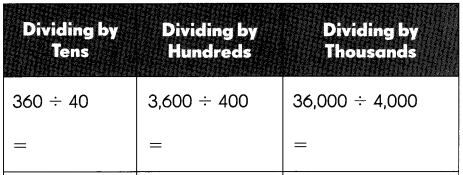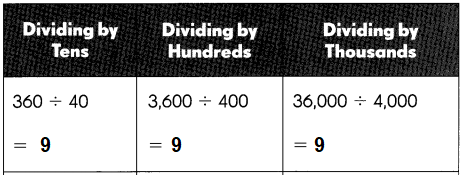Explanation:
Dividing by tens 360 ÷ 40,
= 360÷10÷4,
= 36÷4,
= 9,
= 3600 ÷ 400
Dividing by hundreds 3600÷100÷4,
= 36÷4
= 9,
Dividing by thousands 36000 ÷ 4000,
= 360÷1000÷4,
= 36÷4,
= 9.

Question 27.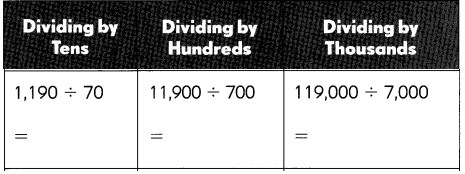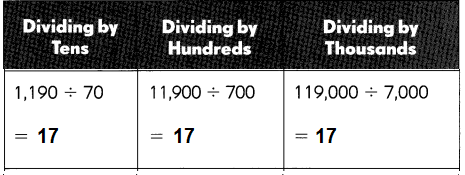Explanation:
Dividing by tens 1,190 ÷ 70,
= 1190 ÷ 10÷ 7
= 119 ÷ 7
= 17,
Dividing by hundreds 11,900 ÷ 700,
= 11,900 ÷ 100÷ 7
= 119 ÷ 7
= 17,
Dividing by thousands 1,19000 ÷ 7000,
= 119000 ÷ 1000÷ 7,
= 119 ÷ 7,
= 17.

Question 28.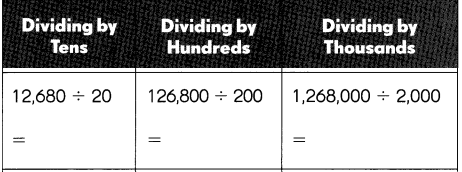634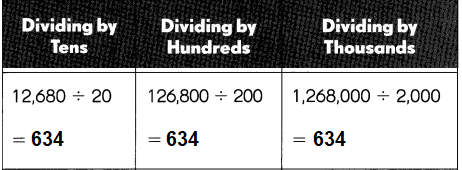Explanation:
Dividing by tens 12,680 ÷ 20,
= 12,680 ÷ 10 ÷ 2,
= 1,268 ÷ 2,
= 634.
Dividing by hundreds 126,800 ÷ 200,
= 126,800÷ 100÷ 2,
= 1,268 ÷ 2,
= 634.
Dividing by thousands 1,268,000 ÷ 2000,
= 1,268,000 ÷ 2,000,
= 12,680 ÷ 1,000 ÷ 2,
=1,268 ÷ 2,
= 634.

Question 29.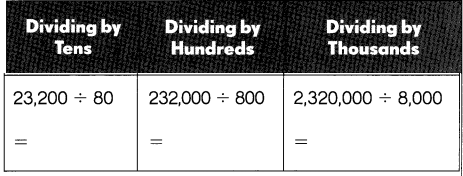290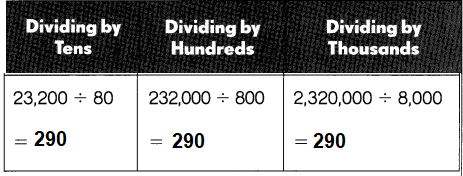Explanation:
Dividing by tens 23,200 ÷ 80,
= 23,200 ÷ 10 ÷ 8,
= 23,20 ÷ 8,
= 290.
Dividing by hundreds 23,2000 ÷ 800,
= 23,2000 ÷ 100 ÷ 8,
= 2320 ÷ 8,
= 290.
Div iding by thousands 2,320,000 ÷ 8000,
= 2,320,000÷ 1000÷ 8,
= 2320 ÷ 8,
= 290.

Complete.

Question 30.
430 ÷ _10_ = 43
10,
Explanation:
Given 430 ÷ 10 = 43.

Question 31.
9,000 ÷ _100_ = 30
300,

Explanation:
Given 9,000 ÷ 300,
=9,000 ÷ 100 ÷ 3,
= 90 ÷ 3 = 30.

Question 32.
49,000 ÷ _1,000__ = 7
7,000,

Explanation:
49,000 ÷ 7,000,
= 49,000 ÷ 1,000 ÷ 7,
= 49 ÷ 7 = 7.

Question 33.
2,400 ÷ _10__ = 120
20,

Explanation:
Given 2,400 ÷ _10__ = 120,
= 2,400 ÷ 120,
= 2,400 ÷ 10 ÷ 12,
= 240 ÷ 12 = 20.

Question 34.
64,000 ÷ _400__ = 160
400,

Explanation:
Given 64,000 ÷ 400,
= 64,000 ÷ 100 ÷ 4,
= 640 ÷ 4 = 160.

Question 35.
85,000 ÷ _5,000__ = 17
5,000,

Explanation:
Given 85,000 ÷ ____ = 17,
= 85,000 ÷ 17 = 5,000.

Estimate each quotient.

Example
6,452 ÷ 27 rounds to 6,000 ÷ 3 = 200

Question 36.
7,865 ÷ 41 rounds to ____ ÷ ___ = ____
7,865 ÷ 41 rounds to 8,000 ÷ 40 = 200,

Explanation:,
Estimate of 7,865 is 8,000,
Estimate of 41 is 40,
So, 8,000 ÷ 40 = 200.

Question 37.
9,125 ÷ 345 rounds to ___ ÷ ___ = ____
9,125 ÷ 345 rounds to 9,000 ÷ 300 = 30,

Explanation:
Estimate of 9,125 is 9,000,
Estimate of 345 is 300,
So, 9,000 ÷ 300 = 30.

Question 38.
9,825 ÷ 206 rounds to ___ ÷ ___ = ____
9,825 ÷ 206 rounds to 10,000 ÷ 200 = 50,

Explanation:
Estimate of 9,825 is 10,000,
Estimate of 206 is 200,
So, 10,000 ÷ 200 = 50.

Question 39.
7,226 ÷ 871 rounds to ___ ÷ ___ = ____
7,226 ÷ 871 rounds to 7200 ÷ 900 = 8,

Explanation:
Estimate of 7,226 is 7,200,
Estimate of 871 is 900,
So, 7,200 ÷ 900 = 8.

Question 40.
5,299 ÷ 49 rounds to ___ ÷ ____ = ____
5,299 ÷ 49 rounds to 5,000 ÷ 50 = 100,

Explanation:
Estimate of 5,299 is 5,000,
Estimate of 49 is 50,
So, 5,000 ÷ 50 = 100.

Question 41.
3,654 ÷ 27 rounds to ___ ÷ ___ = ____
3,654 ÷ 27 rounds to 3,600 ÷ 30 = 120,

Explanation:
Estimate of 3,654 is 3,600,
Estimate of 27 is 30,
So, 3,600 ÷ 30 = 120.

What number can be evenly divided by 3, 7, and 9?
Color the numbers below that match the answers above to find out.